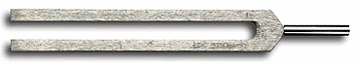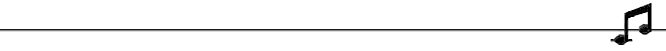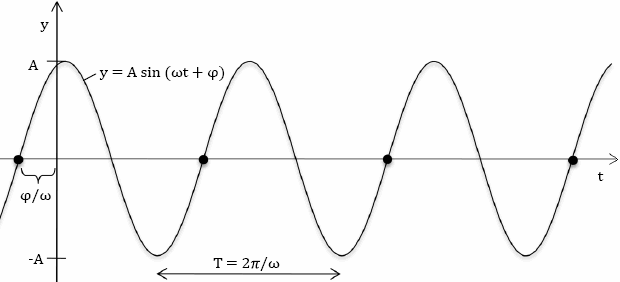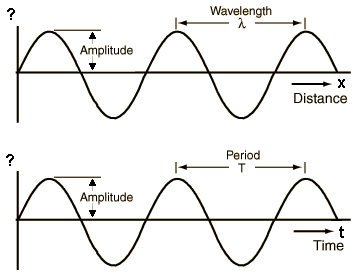Deutsche VersionFrequency  formulaConversion and calculation
Period, cycle duration, periodic time, time T to frequency f,
and frequency f  to cycle duration or period T T = 1 / f and f = 1 / T
hertz to milliseconds and frequency to angular frequency

The only kind of periods meant by people who use this phrase are periods of time, so it's a redundancy. Simply say "time" or "period."Frequency f Hz | Period T ms | ↓ | ↓ | Period T ms | Frequency f Hz |

Fill out the gray box above and click at the calculation bar of the respective column.

Frequency means oscillations (cycles) per second in Hz = hertz = 1/s.
1 second = 1 s = 1000 ms | 1 ms = 0.001 s | 1 µs = 0.000001 s
cps = cycles per secondTo use the calculator, simply enter a value. The calculator works in both directions of the ↔ sign.

 Period = Time Frequency f   Hz ↔ Period T   ms T = 1 / f                              f = 1 / T

Oszilloscope: Input of the boxes (Div.) and timebase (Y) give the frequency.

 Centimeters per period / div. cm Timebase Y ms ↓ Frequency f = 1/T Hz

Formula for period (cycle duration) T

 Physical value symbol unit abbreviation formula Cycle duration T = 1 / f second s T = λ / c Frequency f = 1 / T hertz Hz = 1/s f = c / λ Wavelength λ meter m λ = c / f Wave speed c meter per second m/s c = λ × f

 Frequency formula    f = 1 / T und f = c / λTime conversion - As time goes by

Formulas and equations for frequency and wavelength

 The formula for frequency is: f (frequency) = 1 / T (period). f = c / λ = wave speed c (m/s) / wavelength λ (m). The formula for time is: T (period) = 1 / f (frequency). The formula for wavelength is λ (m) = c / f λ = c / f = wave speed c (m/s) / frequency f (Hz). The unit hertz (Hz) was once called cps = cycles per second.

c = λ × f          λ = c / f = c × T          f = c / λ

Differentiate the Speed of the Medium:
Speed of Sound or Speed of Light

 Choose: Speed of sound in air at a temperature of 20°C: c = 343 m/s or speed of radio waves and light in a vacuum: c = 299,792,458 m/s.   The propagation speed of electrical signals via optical fiber is about 9/10 the speed of light, that is ≈ 270,000 km/s. The propagation speed of electrical signals via copper cables is about 2/3 the speed of light, that is ≈ 200,000 km/s.   Speed of sound c = 343 m/s also equates to 1235 km/h, 767 mph, 1125 ft/s.

There are four parts to a wave:
wavelength, period, frequency, and amplitude

Changing the frequency (hertz, Hz) does never change the amplitude and vice versaThe Angular Frequency is ω = 2π × f

 Given is the equation: y = 50 sin (5000 t) Determine the frequency and the amplitude. Answer: The amplitude is 50 and ω = 5000. So the frequency is f = 1/T = ω / 2 π = 795.77 Hz.

 To use the calculator, simply enter a value. The calculator works in both directions of the ↔ sign.

 Frequency f Hz ↔ Angular Frequency ω rad/s ω = 2π × f                              f = ω / 2π

Conversion: Frequency to wavelength and vice versaSine wave or sinusoid and the period T

 In physics and electrical engineering for the sinusoidal process is often used the angular frequency ω instead of the frequency f. The speed or frequency of revolution is a size at - preferably mechanical - rotating movements indicating the frequency of revolutions. For example, it is an essential feature for engines. It will be given in 1/min, as revolutions per minute, or as in rpm.The y axis shows the sound pressure p (sound pressure amplitude). If the graph shows at the x axis the time t, we see the period T = 1 / f. If the graph shows at the x axis the distance d, we see the wavelength λ. The largest deflection or elongation is referred to as amplitude a.

 The amplitude has absolutely nothing to do with the frequency ... also nothing with the wavelength.

● Wave Graphs ●

 Waves may be graphed as a function of time or distance. A single frequency wave will appear as a sine wave (sinosoid) in either case. From the distance graph the wavelength may be determined. From the time graph, the period and frequency can be obtained. From both together, the wave speed can be determined. Source: http://hyperphysics.phy-astr.gsu.edu/hbase/sound/wavplt.htmlIn acoustics an expression for a sine wave is written in the form y = A sin (2 π f T + φ). Where ω = 2 π f and A is the amplitude and where f is the frequency of the wave measured in hertz. Comparing the mathematical form y = A sin (B T + φ): With this acoustical form we see that | B | = 2 π f. Hence we have the frequency f = | B | / 2 π and the period T = 2 π / | B | = 1 / f.

 SI multiples for hertz (Hz) Value Symbol Name Value Symbol Name 10−1 Hz dHz decihertz 101 Hz daHz decahertz 10−2 Hz cHz centihertz 102 Hz hHz hectohertz 10−3 Hz mHz millihertz 103 Hz kHz kilohertz 10−6 Hz µHz microhertz 106 Hz MHz megahertz 10−9 Hz nHz nanohertz 109 Hz GHz gigahertz 10−12 Hz pHz picohertz 1012 Hz THz terahertz 10−15 Hz fHz femtohertz 1015 Hz PHz petahertz 10−18 Hz aHz attohertz 1018 Hz EHz exahertz 10−21 Hz zHz zeptohertz 1021 Hz ZHz zettahertz 10−24 Hz yHz yoctohertz 1024 Hz YHz yottahertz Common prefixed units are in bold face.

A typical question: What is the relationship between wavelength, temperature, and frequency?

 Explain the relationship between distance, time, and frequency in determining wavelength or: What is the equation with frequency, distance, and time? Speed = distance / time Speed = wavelength × frequency therefore Wavelength × frequency = distance / time therefore Wavelength = distance / (time × frequency)Masterclock calculator (clock rate)

 To use the calculator, simply enter a value. The calculator works in both directions of the ↔ sign.

 Reference wordclock 48.0 kHz  Piano tuning f  Hz ↔ Reference frequency 440 Hz  Studio wordclock fs  kHz

 Reference wordclock 44.1 kHz  Piano tuning f  Hz ↔ Reference frequency 440 Hz  Studio wordclock fs  kHzCalculator with reference frequency

 Reference wordclock kHz Reference frequency Hz Piano tuning f Hz ↓ Studio wordclock fs kHz Interval deviation cent

For downward tuning the reference frequency and piano tuning can be changed.

100 cent is equivalent to a semitone (halftone).